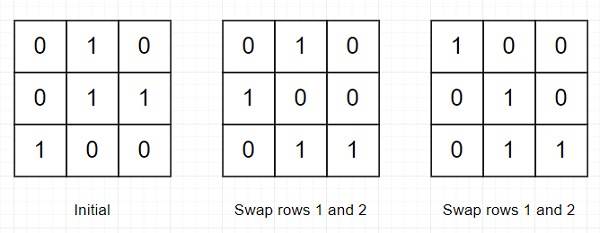# Program to find minimum swaps to arrange a binary grid using Python

Suppose we have a n x n binary matrix. We can perform an operation on it like, at one step we select two adjacent rows and swap them. We have to count number of minimum swaps required, so that all nodes above the major diagonal of the matrix is 0. If there is no such solution, then return -1.

So, if the input is like

 0 1 0 0 1 1 1 0 0

then the output will be 2 because −To solve this, we will follow these steps:

• n := row count of matrix

• m := make an array of size n and fill with n

• for i in range 0 to n - 1, do

• for j in range n-1 to 0, decrease by 1, do

• if matrix[i, j] is same as 1, then

• m[i] := n-j-1

• come out from the loop

• t := 0, ans := 0

• for i in range 0 to n - 1, do

• t := t + 1

• flag := False

• for j in range i to n - 1, do

• if m[j] >= n-t, then

• ans := ans + j-i

• flag := True

• come out from loop

• if flag is false, then

• return -1

• update m[from index i+1 to j] by m[from index i to j-1]

• return ans

Let us see the following implementation to get better understanding −

## Example

Live Demo

def solve(matrix):
n = len(matrix)
m = [n] * n
for i in range(n):
for j in range(n-1,-1,-1):
if matrix[i][j] == 1:
m[i] = n-j-1
break
t,ans = 0,0
for i in range(n):
t += 1
flag = False
for j in range(i,n):
if m[j] >= n-t:
ans += j-i
flag = True
break
if not flag: return -1
m[i+1:j+1] = m[i:j]
return ans
matrix = [[0,1,0],[0,1,1],[1,0,0]]
print(solve(matrix))

## Input

[[0,1,0],[0,1,1],[1,0,0]]

## Output

2